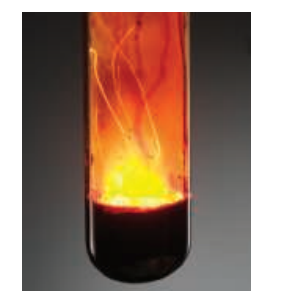×
Get Full Access to Chemistry: The Central Science - 14 Edition - Chapter 3 - Problem 3.69
Get Full Access to Chemistry: The Central Science - 14 Edition - Chapter 3 - Problem 3.69

×

# ?A piece of aluminum foil $$1.00 \mathrm{~cm}^{2}$$ and 0.550-mm thick is allowed to react with bromine to form aluminum bromide.ISBN: 9780134414232 1274

## Solution for problem 3.69 Chapter 3

Chemistry: The Central Science | 14th Edition

• Textbook Solutions
• 2901 Step-by-step solutions solved by professors and subject experts
• Get 24/7 help from StudySoup virtual teaching assistantsChemistry: The Central Science | 14th Edition

4 5 1 288 Reviews
16
1
Problem 3.69

A piece of aluminum foil $$1.00 \mathrm{~cm}^{2}$$ and 0.550-mm thick is allowed to react with bromine to form aluminum bromide.(a) How many moles of aluminum were used? (The density of aluminum is $$2.699 \mathrm{~g} / \mathrm{cm}^{3}$$ .)

(b) How many grams of aluminum bromide form, assuming the aluminum reacts completely?

Text Transcription:

1.00 cm 2

2.699 g/cm 3

Step-by-Step Solution:

Step 1 of 5) How many moles of aluminum were used (The density of aluminum is 2.699 g/cm3 .)(b) How many grams of aluminum bromide form, assuming the aluminum reacts completelyThe First Law of Thermodynamics Energy comes in many different forms, including kinetic energy, which we encountered in Chapter 1, and various types of potential energy. You don’t have to look far to find processes that involve conversions from one form of energy to another. Dropping a stone down a deep well converts gravitational potential energy into kinetic energy. Heating your house by reacting natural gas with oxygen converts chemical energy into heat. In both cases—and every other one you can imagine—energy can be converted from one form to another, but it is neither created nor destroyed. This observation, one of the most important in all of science, is known as the first law of thermodynamics. To apply this law quantitatively we need to divide the universe into a finite system of interest to us, and define the energy of that system more precisely. We explore these concepts next.

Step 2 of 2

##### ISBN: 9780134414232

This full solution covers the following key subjects: . This expansive textbook survival guide covers 29 chapters, and 2820 solutions. The answer to “?A piece of aluminum foil $$1.00 \mathrm{~cm}^{2}$$ and 0.550-mm thick is allowed to react with bromine to form aluminum bromide. (a) How many moles of aluminum were used? (The density of aluminum is $$2.699 \mathrm{~g} / \mathrm{cm}^{3}$$ .) (b) How many grams of aluminum bromide form, assuming the aluminum reacts completely?Text Transcription:1.00 cm 22.699 g/cm 3” is broken down into a number of easy to follow steps, and 56 words. Chemistry: The Central Science was written by and is associated to the ISBN: 9780134414232. The full step-by-step solution to problem: 3.69 from chapter: 3 was answered by , our top Chemistry solution expert on 10/03/18, 06:29PM. This textbook survival guide was created for the textbook: Chemistry: The Central Science, edition: 14. Since the solution to 3.69 from 3 chapter was answered, more than 238 students have viewed the full step-by-step answer.

## Discover and learn what students are asking

Calculus: Early Transcendental Functions : The Cross Product of Two Vectors in Space
?In Exercises 1-6, find the cross product of the unit vectors and sketch your result. $$\mathbf{j} \times \mathbf{k}$$

Unlock Textbook Solution

?A piece of aluminum foil $$1.00 \mathrm{~cm}^{2}$$ and 0.550-mm thick is allowed to react with bromine to form aluminum bromide.